Next: 2.5 Area Under the Up: 2. Some Area Calculations Previous: 2.3 The Area Under   Index

# 2.4 Finite Geometric Series

For each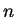in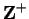let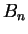denote the box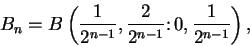and let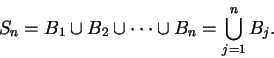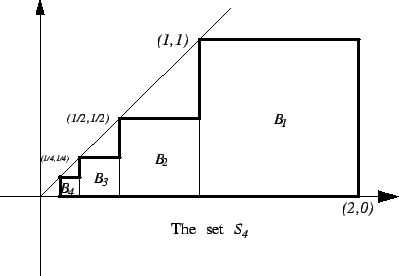I want to find the area of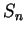. I have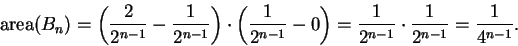Since the boxes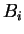intersect only along their boundaries, we have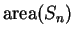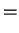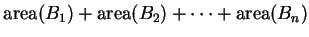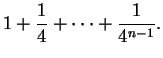(2.20)

Thus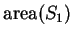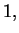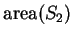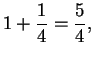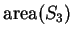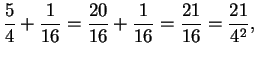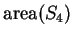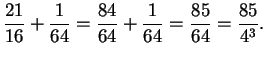(2.21)

You probably do not see any pattern in the numerators of these fractions, but in fact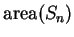is given by a simple formula, which we will now derive.

2.22   Theorem (Finite Geometric Series.) Let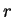be a real number such that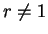. Then for all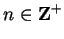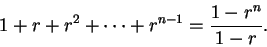(2.23)

Proof: Let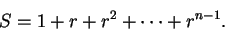Then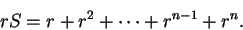Subtract the second equation from the first to get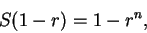and thus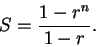Remark: Theorem 2.22 is very important, and you should remember it. Some people find it easier to remember the proof than to remember the formula. It would be good to remember both.

If we let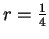in (2.23), then from equation (2.20) we obtain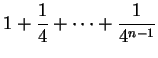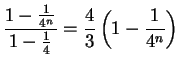(2.24)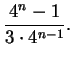As a special case, we have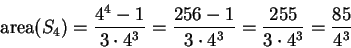which agrees with equation (2.21).

2.25   Entertainment (Pine Tree Problem.) Let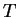be the subset of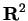sketched below: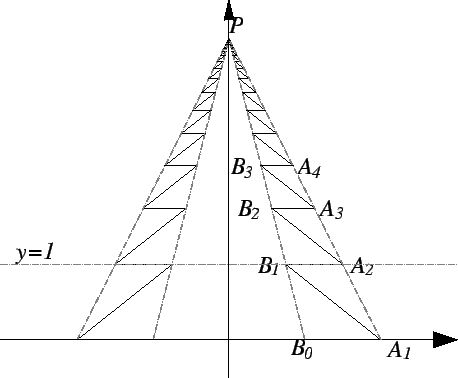Here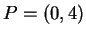,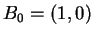,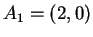, and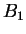is the point where the line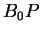intersects the line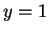. All of the points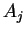lie on the line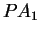, and all of the points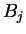lie on the line. All of the segments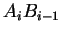are horizontal, and all segments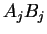are parallel to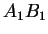. Show that the area ofis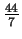. You will probably need to use the formula for a geometric series.

2.26   Exercise.
(a) Find the number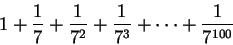accurate to 8 decimal places.

(b) Find the number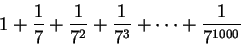accurate to 8 decimal places.

(You may use a calculator, but you can probably do this without using a calculator.)

2.27   Exercise. A Let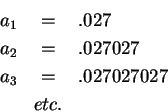Use the formula for a finite geometric series to get a simple formula for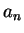. What rational number should the infinite decimal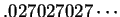represent? Note that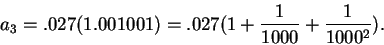The Babylonians[45, page 77] knew that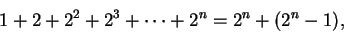(2.28)

i.e. they knew the formula for a finite geometric series when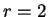.

Euclid knew a version of the formula for a finite geometric series in the case whereis a positive integer.

Archimedes knew the sum of the finite geometric series when. The idea of Archimedes' proof is illustrated in the figure.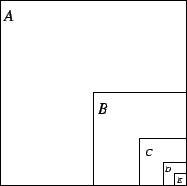If the large square has side equal to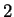, then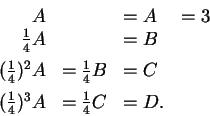Hence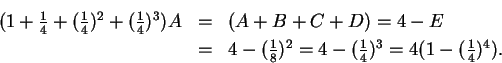i.e.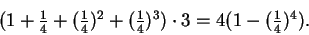For the details of Archimedes' argument see [2, pages 249-250].

2.29   Exercise. Explain why formula (2.28) is a special case of the formula for a finite geometric series.Next: 2.5 Area Under the Up: 2. Some Area Calculations Previous: 2.3 The Area Under   Index
Ray Mayer 2007-09-07